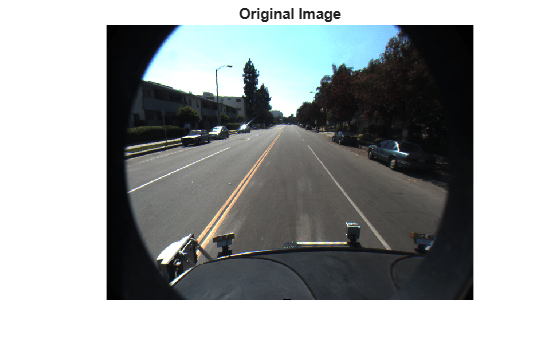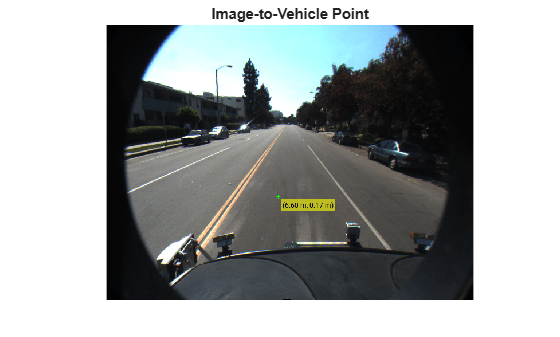Main Content

# vehicleToImage

Convert vehicle coordinates to image coordinates

## Syntax

``imagePoints = vehicleToImage(monoCam,vehiclePoints)``

## Description

example

````imagePoints = vehicleToImage(monoCam,vehiclePoints)` converts [x y] or [x y z] vehicle coordinates to [x y] image coordinates by applying a projective transformation. The monocular camera object, `monoCam`, contains the camera parameters.```

## Examples

collapse all

Create a forward-facing monocular camera sensor mounted on an ego vehicle. Examine an image captured from the camera and determine locations within the image in both vehicle and image coordinates.

Set the intrinsic parameters of the camera. Specify the focal length, the principal point of the image plane, and the output image size. Units are in pixels. Save the intrinsics as a `cameraIntrinsics` object.

```focalLength = [800 800]; principalPoint = [320 240]; imageSize = [480 640]; intrinsics = cameraIntrinsics(focalLength,principalPoint,imageSize);```

Specify the position of the camera. Position the camera 2.18 meters above the ground with a 14-degree pitch toward the ground.

```height = 2.18; pitch = 14;```

Define a monocular camera sensor using the intrinsic camera parameters and the position of the camera. Load an image from the camera.

```sensor = monoCamera(intrinsics,height,'Pitch',pitch); Ioriginal = imread('road.png'); figure imshow(Ioriginal) title('Original Image')```Determine the image coordinates of a point 10 meters directly in front of the camera. The X-axis points forward from the camera and the Y-axis points to the left.

```xyVehicleLoc1 = [10 0]; xyImageLoc1 = vehicleToImage(sensor,xyVehicleLoc1)```
```xyImageLoc1 = 1×2 320.0000 216.2296 ```

Display the point on the image.

```IvehicleToImage = insertMarker(Ioriginal,xyImageLoc1); IvehicleToImage = insertText(IvehicleToImage,xyImageLoc1 + 5,'10 meters'); figure imshow(IvehicleToImage) title('Vehicle-to-Image Point')```Determine the vehicle coordinates of a point that lies on the road surface in the image.

```xyImageLoc2 = [300 300]; xyVehicleLoc2 = imageToVehicle(sensor,xyImageLoc2)```
```xyVehicleLoc2 = 1×2 6.5959 0.1732 ```

The point is about 6.6 meters in front of the vehicle and about 0.17 meters to the left of the vehicle center.

Display the vehicle coordinates of the point on the image.

```IimageToVehicle = insertMarker(Ioriginal,xyImageLoc2); displayText = sprintf('(%.2f m, %.2f m)',xyVehicleLoc2); IimageToVehicle = insertText(IimageToVehicle,xyImageLoc2 + 5,displayText); figure imshow(IimageToVehicle) title('Image-to-Vehicle Point')```## Input Arguments

collapse all

Monocular camera parameters, specified as a `monoCamera` object.

Vehicle points, specified as an M-by-2 or M-by-3 matrix containing M number of [x y] or [x y z] vehicle coordinates.

## Output Arguments

collapse all

Image points, returned as an M-by-2 matrix containing M number of [x y] image coordinates.

## See Also

### Functions

Introduced in R2017a

## Support

#### Implementing an Adaptive Cruise Controller with Simulink

Download technical paper